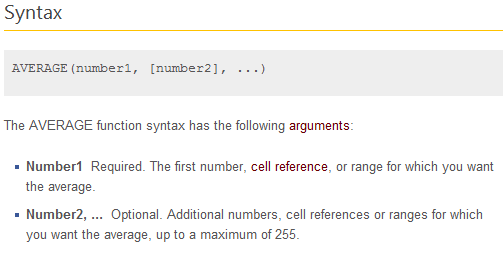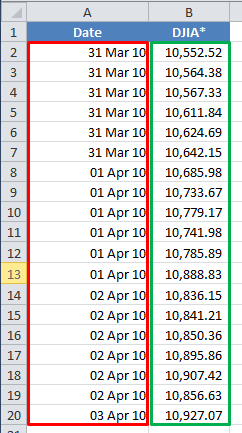# Formula Forensics 016. Suzannes DJIA Average

Last week Suzanne asked a question over at the Excel Hero Academy.

I was trying to calculate the average DJIA # by month. I had the data by day so tried various combos (none of which worked (:-

Suzanne’s Formula: =AVERAGE(\$A\$2:\$A\$20=31/3/2010, B2:B20)

So today we will pull Suzanne’s Formula apart to see what’s inside and why it didn’t work.

And then we’ll go on to recommend a solution.

## Suzanne’s Formula

As usual we will work through this formula using a sample file for you to follow along. Download Here.

Suzanne’s formula uses a simple Excel Average( ) function.

=AVERAGE(\$A\$2:\$A\$20=31/3/2010, B2:B20)

The Average Function has the syntaxSo Average will sum up the numbers in the function or supplied Ranges and then divide by the number of entries or cells in the range

If we look at Suzanne’s formula =AVERAGE(\$A\$2:\$A\$20=31/3/2010, B2:B20)

We can see that it has two ranges

=AVERAGE(\$A\$2:\$A\$20=31/3/2010, B2:B20)Range 1: \$A\$2:\$A\$20=31/3/2010

Range 2: B2:B20

Lets look at the first range

Range 1: \$A\$2:\$A\$20=31/3/2010

In a blank cell say E12 enter =\$A\$2:\$A\$20=31/3/2010 then press F9 instead of Enter

Excel will return ={FALSE; FALSE; FALSE; FALSE; FALSE; FALSE; FALSE; FALSE; FALSE; FALSE; FALSE; FALSE; FALSE; FALSE; FALSE; FALSE; FALSE; FALSE; FALSE}

This is telling us a few things:

Firstly that none of the cells in the range match the date 31/3/2010

But we can see that the first 6 items all match.

So maybe it is the format of the date?

In a 2nd blank cell say E13 enter =\$A\$2:\$A\$20=”31/3/2010” then press F9 instead of Enter

Excel will return ={FALSE; FALSE; FALSE; FALSE; FALSE; FALSE; FALSE; FALSE; FALSE; FALSE; FALSE; FALSE; FALSE; FALSE; FALSE; FALSE; FALSE; FALSE; FALSE}

Once again Excel is not finding any matches.

In a 3rd blank cell say cell E14 enter =\$A\$2:\$A\$20=Date(2010,3,31) then press F9 instead of Enter

Excel will return ={TRUE; TRUE; TRUE; TRUE; TRUE; TRUE; FALSE; FALSE; FALSE; FALSE; FALSE; FALSE; FALSE; FALSE; FALSE; FALSE; FALSE; FALSE; FALSE}

Bingo, We now have 6 matches. Suzanne was using a bad syntax for matching the dates.

Now lets look at the second range

Range 2: B2:B20

In a blank cell say E16 enter =B2:B20 then press F9 instead of Enter

Excel will return = {10552.52; 10564.38; 10567.33; 10611.84; 10624.69; 10642.15; 10685.98; 10733.67; 10779.17; 10741.98; 10785.89; 10888.83; 10836.15; 10841.21; 10850.36; 10895.86; 10907.42; 10856.63; 10927.07}

It is a list of all the numbers between B2 and B20, they have all been included.

=AVERAGE(\$A\$2:\$A\$20=31/3/2010, B2:B20)

We can now see that the two ranges will translate to

=AVERAGE({FALSE; FALSE; FALSE; FALSE; FALSE; FALSE; FALSE; FALSE; FALSE; FALSE; FALSE; FALSE; FALSE; FALSE; FALSE; FALSE; FALSE; FALSE; FALSE},{10552.52; 10564.38; 10567.33; 10611.84; 10624.69; 10642.15; 10685.98; 10733.67; 10779.17; 10741.98; 10785.89; 10888.83; 10836.15; 10841.21; 10850.36; 10895.86; 10907.42; 10856.63; 10927.07})

So in E18 now enter =AVERAGE({FALSE; FALSE; FALSE; FALSE; FALSE; FALSE; FALSE; FALSE; FALSE; FALSE; FALSE; FALSE; FALSE; FALSE; FALSE; FALSE; FALSE; FALSE; FALSE},{10552.52; 10564.38; 10567.33; 10611.84; 10624.69; 10642.15; 10685.98; 10733.67; 10779.17; 10741.98; 10785.89; 10888.83; 10836.15; 10841.21; 10850.36; 10895.86; 10907.42; 10856.63; 10927.07})

Excel returns 10752.27, which is the incorrect answer that we also see in Suzanne’s formula in E4

Excel, true to form has done exactly what we asked it to do, except that we have asked it to do the wrong thing.

## So How Do We Average the DJIA Values

How do we average the DJIA values where the date is equal to 31/3/2010.

We saw that in the 3rd Blank cell E14 that when we entered =\$A\$2:\$A\$20=Date(2010,3,31), Excel responded with an array of True/False values, where the dates matched our value.

We can use a small modification to this to just extract the exact dates and ignore the rest.

If we simply use this formula in conjunction with an If( ) function

IF(\$A\$2:\$A\$20=DATE(2010,3,31), \$B\$2:\$B\$20)

In the If function if the cells in A2:A20 match the Date of 31/3/2010 then Excel will return the True value or the corresponding value from B2:B20

To test this in a Blank cell say E20 enter =IF(\$A\$2:\$A\$20=DATE(2010,3,31),\$B\$2:\$B\$20) press F9

Excel responds with an array ={10552.52; 10564.38; 10567.33; 10611.84; 10624.69; 10642.15; FALSE; FALSE; FALSE; FALSE; FALSE; FALSE; FALSE; FALSE; FALSE; FALSE; FALSE; FALSE; FALSE}

Excel has returned an array of the first 6 values from B2:B20 which correspond to the 6 matching cells from A2:A20 and the returned False for the remaining cells from B8:B20

If we include this array in an Average Function we should be home.

In Cell E6 enter the formula =AVERAGE(IF(\$A\$2:\$A\$20=DATE(2010,3,31),\$B\$2:\$B\$20)) Press Ctrl Shift Enter instead of Enter

Excel responds with 10,593.8183 matching our manual calculations in cell E2.

## Why Didn’t we put a False Value in the If statement ?

In Cell E22 enter the formula =IF(\$A\$2:\$A\$20=DATE(2010,3,31),\$B\$2:\$B\$20,0) Press Ctrl Shift Enter instead of Enter

Excel responds with an array ={10552.52; 10564.38; 10567.33; 10611.84; 10624.69; 10642.15; 0; 0; 0; 0; 0; 0; 0; 0; 0; 0; 0; 0; 0}

This is the first 6 values from Column B matching our criteria and the 13 entries of 0 where it doesn’t match

If we sum these numbers up we get 63,562.91

If we divide 63,562.91 by 19 (the number of values) we get an average of 3,345.42

We can see that excel has included the 13, “0” values in the average as numbers hence reducing the average, where it has ignored the 13 Falses in our previous formula to just average the 6 matching values.

## An Alternative Solution

Excel has a handy function which will solve this problem for Suzanne without using an Array Formula?

It’s called Averageifs( ) and it is new to Excel 2007 and above.

Suzannes problem is simply solved with:

`=AVERAGEIFS(B2:B20, A2:A20, DATE(2010,3,31))`

Which says, Average column B2:B20 where Column A2:A20 = 31 March 2010

Benefits of Averageifs( )

The two benefits of using Averageifs( )

1. It isn’t array entered

2. It can have multiple other conditions added to it

Shortcoming of Averageifs( )

The main shortcoming of Averageifs( )

1. It is not available in Excel before 2007

## Formula Forensics “The Series”

Formula Forensic Series

## Formula Forensics Needs Your Help

I urgently need more ideas for future Formula Forensics posts and so I need your help.

If you have a neat formula that you would like to share and explain, try putting pen to paper and draft up a Post like above or;

If you have a formula that you would like explained but don’t want to write a post also send it to Chandoo or Hui.

ps: Happy Birthday Jhuvy !Hello Awesome...

My name is Chandoo. Thanks for dropping by. My mission is to make you awesome in Excel & your work. I live in Wellington, New Zealand. When I am not F9ing my formulas, I cycle, cook or play lego with my kids. Know more about me.

Thank you and see you around.

### Related articles:

 Written by Hui... Tags: average(), averageifs, date, if() Home: Chandoo.org Main Page ? Doubt: Ask an Excel Question

### 8 Responses to “Formula Forensics 016. Suzannes DJIA Average”

1. [...] Cara Mengkonversi Latitude dan longitude pada Google Maps ke Formula Array Pada Microsoft ExcelMicrosoft Excel 2010 Complete (Shelly Cashman Series) (9780538750059) Gary B. Shelly, Jeffrey J. QuasneyMicrosoft Excel 2010 Comprehensive (Shelly Cashman) (9781439079010) Gary B. Shelly, Jeffrey J. QuasneyLearn Excel 2010 – “Consolidate and Lookup”: Podcast #1535Total Excel ConverterTotal Excel Converter 2.9 keygen key download activate serialHow To: Add Excel Files To BloggerMicrosoft Office Excel 2007 Complete Concepts and Techniques (Shelly Cashman Series) (9781418843434) Gary B. Shelly, Thomas J. Cashman, Jeffrey J. QuasneyVisual Basic for ApplicationsHow to average the DJIA over selected days [...]

2.yashwanth says:

Hi chandoo,
i have read some of your blogs..to be frank, i was in awe after seeing your knowledge in Excel, I got calls from IIMs and planning to join IIM L or FMS. I have heard excel is very useful in B school, i am trying to learn it from your blogs. I want to improve my MS Office skills also like powerpoint, word and all... can you tell me any resources or books from where i can master MS office. I have seen your recommended list for MS Excel and purchased a few....but for other Office softwares, i am a bit confuesd....can you kindly help me with that.....thanks in advance

•Chandoo says:

@Yahswanth.. thanks for your comments. I do not recommend any site or book for MS word as you can pick most of the required stuff after writing a few assignments using it.

For Powerpoint, try the books

- Design book for non-designers
- Presentation Zen
- Slide:ology

You can also watch excellent presentations on ted.com or youtube.com. You can also get some good slide ideas & design inspiration from slideshare.net.

3.Jason says:

An alternative for pre-Excel 2007 could be to combine a SUMIF divided by COUNTIF using the same criteria in both to create your mean value, e.g.
=SUMIF(A2:A20, DATE(2010,3,31), B2:B20) / COUNTIF(A2:A20, DATE(2010,3,31))

This would be backward compatible without relying on an array formula (which I prefer to do).

If the criteria is likely to change then you can link both parts to a seperate cell that contains that criteria.

4.Agustín García (Freelancer Excel VBA) says:

Saludos a todos, y felicitar al Administrador de este blog que el contenido es excelente, tratando de aportar con una alternativa mas seria utilizar la función "FECHANUMERO", aplicado al archivo, adjunto el archivo con el ejemplo en la celda D10 y E10
http://dl.dropbox.com/u/34130960/Blog/Blog_freelancermicrosoftexcel/Formulas/Suzannes-Averages.xls

En esta publicación explico la función:
http://freelancermicrosoftexcel.blogspot.com/2011/10/funciones-de-excel-fechanumero-fecha-y.html

saludos cordiales.

"Greetings to all, and congratulate the Administrator of this blog that the content is excellent, trying to provide a cheaper alternative would use the "DATEVALUE" applied to the file, attach the file with the example in cell D10 and E10
http://dl.dropbox.com/u/34130960/Blog/Blog_freelancermicrosoftexcel/Formulas/Suzannes-Averages.xls

In this paper explain the function:
http://freelancermicrosoftexcel.blogspot.com/2011/10/funciones-de-excel-fechanumero-fecha-y.html

best regards."

5.Crisu says:

Hello,
from what I remember Excel stores dates as numbers (dd-mm-rrrr etc is only a format, what we see), so I think that that one should work too:

=AVERAGE(IF(\$A\$2:\$A\$20=40268,\$B\$2:\$B\$20))

Another example is pointing Excel to a cell instead of typing the date manually in the formula, like this:

=AVERAGE(IF(\$A\$2:\$A\$20=A2,\$B\$2:\$B\$20))
(assuming A2 has the date we're interested in).

But generally these are only "cosmetic" changes 😉
Cheers.

6.BBohr says:

Suzanne’s original request involved taking the DJIA average by month. The formulas presented here don’t take the average by month, but rather do so by date. Errors will arise if the average must include data with different dates. For example, suppose the data in A2 of the original file read "30 Mar 10.” The formulas would report an incorrect average because this datum would have been omitted.

I present an alternative that takes the average by month. My typical approach for this sort of problems is to use a helper column that lists the date’s month and then use an averageif. Here, however, I use another approach that doesn’t require the helper column.

=SUMPRODUCT((3=MONTH(\$A\$2:\$A\$20))*\$B\$2:\$B\$20)/SUMPRODUCT(--(3=MONTH(\$A\$2:\$A\$20)))

This formula calculates the average for March (i.e. “3”) based on the cell references in the original file posted. I’d be happy to learn about a more elegant solution than the one I present here.

7.Sandeep Lahoti says:

Avrage if can be used if user didn't had Excel 2007 and above

Formula for the example shall be :

=AVERAGEIF(A2:B20,DATE(2010,3,31),B2:B20)

 « 75 Excel Speeding up Tips Shared by YOU! [Speedy Spreadsheet Week] Comprehensive Guide to VLOOKUP & Other Lookup Formulas »

### Get FREE Excel & Power-BI Newsletter

One email per week with Excel and Power BI goodness. Join 100,000+ others and get it free.